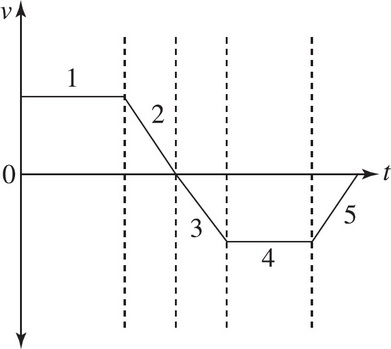# AP Physics 1: Kinematics Multiple-Choice Practice Questions

### Test Information10 questions18 minutes1. In section 5 of the velocity-versus-time graph, the object is

2. A baseball is thrown straight upward. What is the ball's acceleration at its highest point?

3. How long would it take a car, starting from rest and accelerating uniformly in a straight line at 5 m/s2, to cover a distance of 200 m ?

4. A rock is dropped off a cliff and strikes the ground with an impact velocity of 30 m/s. How high was the cliff?

5. A stone is thrown horizontally with an initial speed of 10 m/s from a bridge. Assuming that air resistance is negligible, how long would it take the stone to strike the water 80 m below the bridge?

6. A soccer ball, at rest on the ground, is kicked with an initial velocity of 10 m/s at a launch angle of 30°. Calculate its total flight time, assuming that air resistance is negligible.

7. A stone is thrown horizontally with an initial speed of 30 m/s from a bridge. Find the stone's total speed when it enters the water 4 seconds later, assuming that air resistance is negligible.

8. Which one of the following statements is true concerning the motion of an ideal projectile launched at an angle of 45° to the horizontal?

9. A stone is thrown vertically upward with an initial speed of 5 m/s. What is the velocity of the stone 3 seconds later?

10. A car travelling at a speed of v0 applies its brakes, skidding to a stop over a distance of x m. Assuming that the deceleration due to the brakes is constant, what would be the skidding distance of the same car if it were traveling with twice the initial speed?